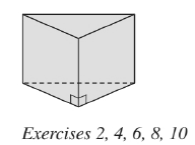Chapter 9.1, Problem 4EElementary Geometry For College St...

7th Edition
Alexander + 2 others
ISBN: 9781337614085

Solutions

Chapter
SectionElementary Geometry For College St...

7th Edition
Alexander + 2 others
ISBN: 9781337614085
Textbook Problem

Consider the triangular prism shown in Exercise 2. a ) How many vertices does it have? b ) How many edges (lateral edges plus base edges) does it have? c ) How many faces (lateral face plus bases) does it have?To determine

a)

To find:

The number of vertices.

Explanation

Calculation:

Given,

The triangular prism has 6 vertices...

To determine

b)

To find:

The number of edges (lateral edges plus base edges).

To determine

c)

To find:

The number of faces (lateral faces plus bases).

Still sussing out bartleby?

Check out a sample textbook solution.

See a sample solution

The Solution to Your Study Problems

Bartleby provides explanations to thousands of textbook problems written by our experts, many with advanced degrees!

Get Started

In Exercises 75-98, perform the indicated operations and/or simplify each expression. 89. (x + 2y)2

Applied Calculus for the Managerial, Life, and Social Sciences: A Brief Approach

6. Compute .

Mathematical Applications for the Management, Life, and Social Sciences

Evaluate 1m when m=3.

Calculus: An Applied Approach (MindTap Course List)

Construct a drawing of the fourth hexagonal number.

Mathematical Excursions (MindTap Course List)

Find the remaining trigonometric ratios. 34. csc=43,322

Single Variable Calculus: Early Transcendentals